Nathan Smith

•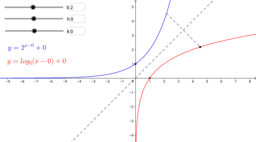Exponential and Logarithmic Functions

Activity

Nathan Smith

•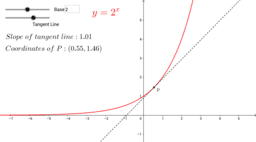The Natural Exponential Function

Activity

Nathan Smith

•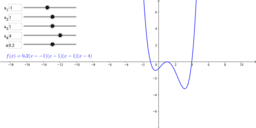Fourth-Degree Polynomials

Activity

Nathan Smith

•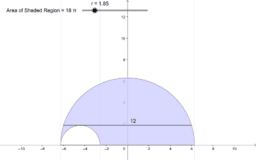Semicircles with a Constant Chord

Activity

Nathan Smith

•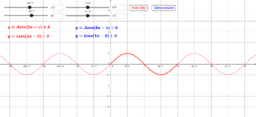Sine and Cosine | y = A sin (bx - c) + k

Activity

Nathan Smith

•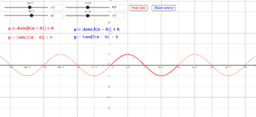Sine and Cosine | y = A sin [b(x - h)] + k

Activity

Nathan Smith

•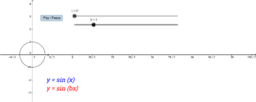y = sin(bx)

Activity

Nathan Smith

•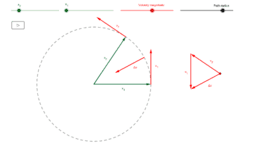Centripetal Acceleration

Activity

Nathan Smith

•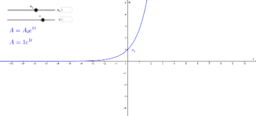Natural Exponential Models

Activity

Nathan Smith

•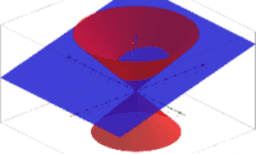Intersection of a Cone and a Plane: The Conic Sections

Activity

Nathan Smith

•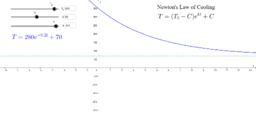Newton's Law of Cooling

Activity

Nathan Smith

•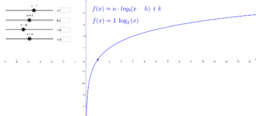Logarithmic Functions

Activity

Nathan Smith

•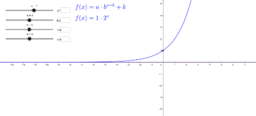Exponential Functions

Activity

Nathan Smith

•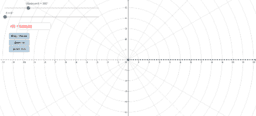Polar Function Grapher

Activity

Nathan Smith

•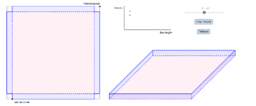Box Formed From a Square

Activity

Nathan Smith

•Bat

Activity

Nathan Smith

•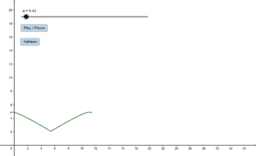Bird

Activity

Nathan Smith

•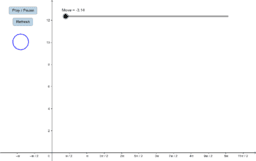Bouncing Ball

Activity

Nathan Smith

•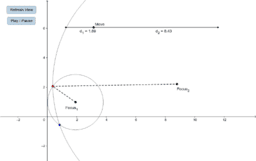Activity

Nathan Smith